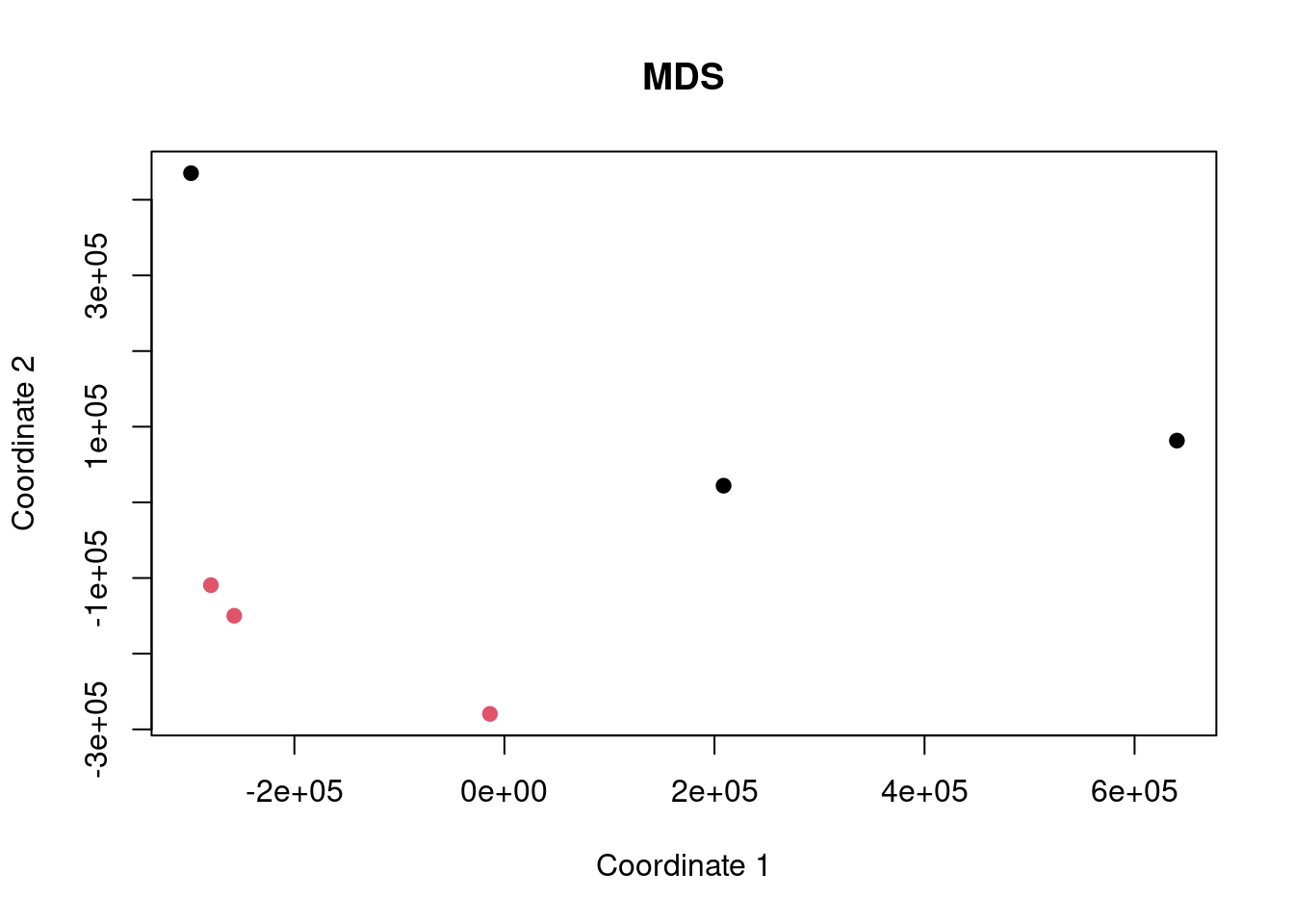## Intro

Here we are performing an analysis of some gene expression data to demonstrate the difference between ORA and FCS methods and to highlight the differences caused by improper background gene set use.

The dataset being used is SRP128998 and we are comparing the cells grown in normal glucose condition (control) to the high glucose condition (case).

Data are obtained from http://dee2.io/

``````suppressPackageStartupMessages({
library("getDEE2")
library("DESeq2")
library("clusterProfiler")
library("mitch")
library("kableExtra")
library("eulerr")
})

dir.create("images")``````
``## Warning in dir.create("images"): 'images' already exists``

## Get expression data and make an MDS plot

I’m using some RNA-seq data looking at the effect of hyperglycemia on hepatocytes.

``````name="SRP128998"
samplesheet <- mdat[grep("SRP128998",mdat\$SRP_accession),]
samplesheet<-samplesheet[order(samplesheet\$SRR_accession),]
samplesheet\$trt<-as.factor(c(1,1,1,1,1,1,0,0,0,0,0,0))
samplesheet\$VPA<-as.factor(c(0,0,0,1,1,1,0,0,0,1,1,1))
s1 <- subset(samplesheet,VPA==0)

s1 %>% kbl(caption = "sample sheet") %>% kable_paper("hover", full_width = F)``````
sample sheet
SRR_accession QC_summary SRX_accession SRS_accession SRP_accession Sample_name GEO_series Library_name trt VPA
475895 SRR6467479 PASS SRX3557428 SRS2830728 SRP128998 GSM2932791 GSE109140 1 0
475896 SRR6467480 PASS SRX3557429 SRS2830730 SRP128998 GSM2932792 GSE109140 1 0
475897 SRR6467481 PASS SRX3557430 SRS2830729 SRP128998 GSM2932793 GSE109140 1 0
475901 SRR6467485 PASS SRX3557434 SRS2830733 SRP128998 GSM2932797 GSE109140 0 0
475902 SRR6467486 PASS SRX3557435 SRS2830734 SRP128998 GSM2932798 GSE109140 0 0
475903 SRR6467487 PASS SRX3557436 SRS2830735 SRP128998 GSM2932799 GSE109140 0 0
``w<-getDEE2("hsapiens",samplesheet\$SRR_accession,metadata=mdat,legacy = TRUE)``
``````## For more information about DEE2 QC metrics, visit
##     https://github.com/markziemann/dee2/blob/master/qc/qc_metrics.md``````
``````x<-Tx2Gene(w)
x<-x\$Tx2Gene

# save the genetable for later
gt<-w\$GeneInfo[,1,drop=FALSE]
gt\$accession<-rownames(gt)

# counts
x1<-x[,which(colnames(x) %in% s1\$SRR_accession)]``````

Here show the number of genes in the annotation set, and those detected above the detection threshold.

``````# filter out lowly expressed genes
x1<-x1[which(rowSums(x1)/ncol(x1)>=(10)),]
nrow(x)``````
``##  39297``
``nrow(x1)``
``##  15635``

Now multidimensional scaling (MDS) plot to show the correlation between the datasets. If the control and case datasets are clustered separately, then it is likely that there will be many differentially expressed genes with FDR<0.05.

``plot(cmdscale(dist(t(x1))), xlab="Coordinate 1", ylab="Coordinate 2", pch=19, col=s1\$trt, main="MDS")``## Differential expression

Now run DESeq2 for control vs case.

``y <- DESeqDataSetFromMatrix(countData = round(x1), colData = s1, design = ~ trt)``
``## converting counts to integer mode``
``y <- DESeq(y)``
``## estimating size factors``
``## estimating dispersions``
``## gene-wise dispersion estimates``
``## mean-dispersion relationship``
``## final dispersion estimates``
``## fitting model and testing``
``````de <- results(y)
de<-as.data.frame(de[order(de\$pvalue),])
rownames(de)<-sapply(strsplit(rownames(de),"\\."),"[[",1)
head(de) %>% kbl() %>% kable_paper("hover", full_width = F)``````
baseMean log2FoldChange lfcSE stat pvalue padj
ENSG00000145050 5839.731 -2.753692 0.1518338 -18.13623 0 0
ENSG00000149131 1346.633 2.161115 0.1427218 15.14215 0 0
ENSG00000044574 124889.027 -2.033391 0.1343040 -15.14021 0 0
ENSG00000128228 1676.368 -2.836358 0.1895729 -14.96183 0 0
ENSG00000179218 78785.663 -2.227516 0.1586383 -14.04148 0 0
ENSG00000090520 6751.044 -2.138112 0.1538129 -13.90073 0 0

Now let’s have a look at some of the charts showing differential expression. In particular, an MA plot and volcano plot.

``````maplot <- function(de,contrast_name) {
sig <-subset(de, padj < 0.05 )
up <-rownames(subset(de, padj < 0.05 & log2FoldChange > 0))
dn <-rownames(subset(de, padj < 0.05 & log2FoldChange < 0))
GENESUP <- length(up)
GENESDN <- length(dn)
DET=nrow(de)
SUBHEADER = paste(GENESUP, "up, ", GENESDN, "down", DET, "detected")
ns <-subset(de, padj > 0.05 )
plot(log2(de\$baseMean),de\$log2FoldChange,
xlab="log2 basemean", ylab="log2 foldchange",
pch=19, cex=0.5, col="dark gray",
main=contrast_name, cex.main=0.7)
points(log2(sig\$baseMean),sig\$log2FoldChange,
pch=19, cex=0.5, col="red")
}

make_volcano <- function(de,name) {